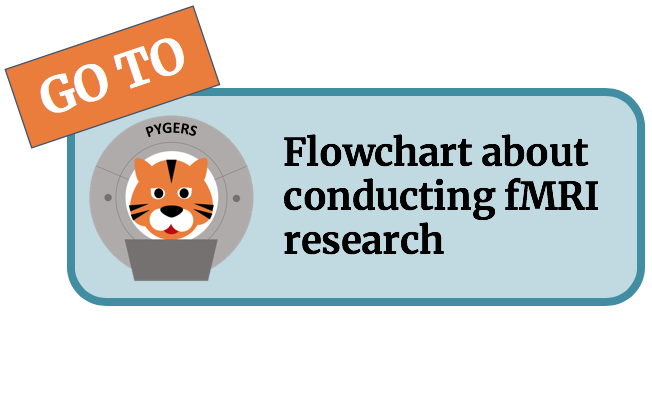# Templates and atlases¶

## A quick glossary¶

• Space: the coordinate space in which templates/atlases live (independent of voxel dimensions!

• Template: the image that the data is actually going to be aligned to

• Atlas: labels applicable to a template in a space

• Parcellations divisions into parcels (usually into labeled sections)
• Labels usually found in ‘.anot’ files

• Registration: transforming an image into the coordinate space of another. In the case of fMRI we register different brains into a common space. Bold data will be in the same resolution that you’re asking for no matter what.

• fs: freesurfer (surface space)

• Resolution: actual pixel dimensions of your data (3x3x3 etc.)

• Asymmetric template: actual hemispheres are not symmetric–otherwise people just mirror hemispheres!!
• Talaraich space is symmetric (mirrored hemispheres)

• Transformations: taking a 4d brain image ( 3D voxels and time) and multiply it by a matrix (affine matrix for linear transformations) that tells you how to rotate and translate your brain image

• Linear Transformations: use a 4x4 affine matrix with the last dimension for time (timeseries) remains untouched.

• Nonlinear Transformations: ANTs uses a previously validated algorithm called Symmetric Normalization to register the brains

## Templates¶

Templates are typically anatomical brain images in a standard space (e.g., MNI space). Many templates are created by averaging anatomical images from a large sample of subjects (e.g., MNI152NLin2009cAsym). Templates are used as target images when spatially normalizing images in a subject-specific space to standard space (e.g., using ANTs). Templates may either be in volumetric or surface space.

Two very common volumetric templates are the MNI152NLin2009cAsym used by default in fMRIPrep and the MNI152NLin6Asym distributed by FSL. Volumetric templates are sometimes resampled to different spatial resolutions, such as 0.5 mm, 1.0 mm, and 2.0 mm isotropic voxels. The most common surface-based template is fsaverage. The spatial resolution of a surface template is determined by the linear divisions of an icosahedron (20-sided convex regular polygon; i.e., a d20 for the D&D folks) according to the following equation: if the number of linear divisions n = 64, you will end up with 20 * n**2 faces (81,920 faces/triangles, or 40,960 per hemisphere) and 2 + 10 * n**2 vertices per hemisphere (40,962). Common fsaverage resolutions are fsaverage (163,842 vertices per hemispheres), fsaverage6 (40,962 vertices per hemisphere), fsaverage5 (10,242 vertices per hemisphere).

## Atlases¶

• MNI atlas used by fmriprep: ICBM 152 Nonlinear atlases version 2009
• Surface space
• For each subject, you will get fsnative space (idiosyncratic number of vertices per hemisphere)

• Standard spaces:
• fsaverage(7) (163,842 vertices per hemisphere)

• fsaverage6 (40,962 vertices per hemisphere)

• fsaverage5 (10,242 vertices per hemisphere)

• The number of vertices is determined by the linear divisions of an icosahedron (20-sided polygon; i.e., a d20 for the D&D folks) according to the following equation: if the number of linear divisions n = 64, you will end up with 20 * n**2 faces (81,920 faces/triangles, or 40,960 per hemisphere) and 2 + 10 * n**2 vertices per hemisphere (40,962).

## Atlases and parcellations¶

This is a type of Atlas where brain regions are clustered and labelled. Here is a list of useful parcellations if you want to select a specific ROI: